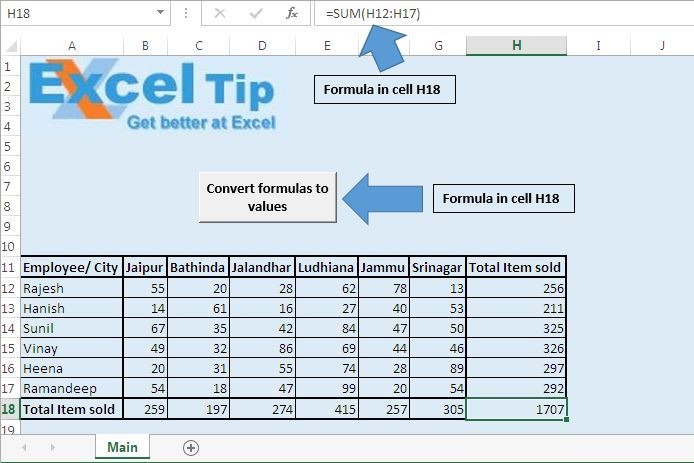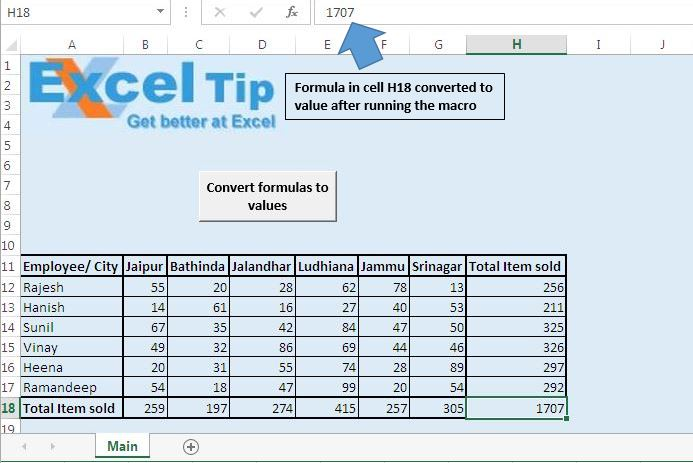Change the formula in the cells in a range to values using VBA in Microsoft Excel

In this article, we will learn how to change the formula in the cells in a range to values using VBA in Microsoft Excel.

Raw data for this example consists of sales data. Sales data contains information regarding number of items sold by employees in different cities. Last row and column contains the sum formula for finding the total number of items sold in a particular city by a particular employee.

We would like to convert all the formulas in the active sheet to values. To convert all the formulas to values, we have created a macro “ChangingFormulasToValue”. This macro can be run by clicking the “Convert formulas to values” button.In the snapshot, one can see formula in H18 cell is converted to value.

Code explanation

Set SourceRng = Range("A1", Range("A1").SpecialCells(xlCellTypeLastCell))

Above code is used to assign all cells starting from cell A1 to the last cell in the workbook as range.

SourceRng.Value = SourceRng.Value

Above code is used to assign the cell value skipping the formula

Option Explicit

Sub ChangingFormulasToValue()

'Declaring variables
Dim SourceRng As Range

'Specify all cells in the active sheet as range
Set SourceRng = Range("A1", Range("A1").SpecialCells(xlCellTypeLastCell))

'Assigning only value of the cell skipping formula of the cell
SourceRng.Value = SourceRng.Value

End Sub

We would love to hear from you, do let us know how we can improve our work and make it better for you. Write to us at info@exceltip.com

1.